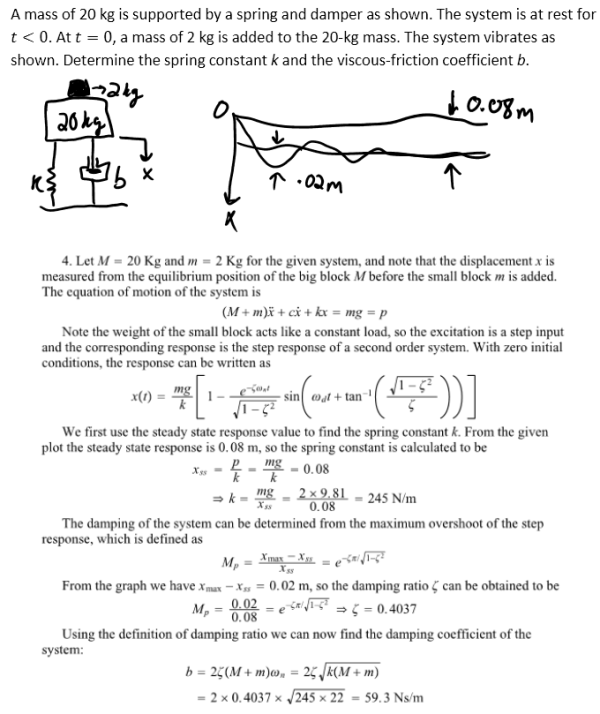A mass of 20 kg is supported by a spring and damper as shown. The system is at rest for t<0. At t=0, a mass of 2 kg is added to the 20-kg mass. The system vibrates as shown. Determine the spring constant k and the viscous-friction coefficient b.A mass of 20 kg is supported by a spring and damper as shown. The system is at rest for t<0. At t=0, a mass of 2 kg is added to the 20-kg mass. The system vibrates as shown. Determine the spring constant k and the viscous-friction coefficient b.

System Dynamics Page 2 dynamics dynamics dynamics dynamics dynamics dynamics dynamics System dynamics Page 3